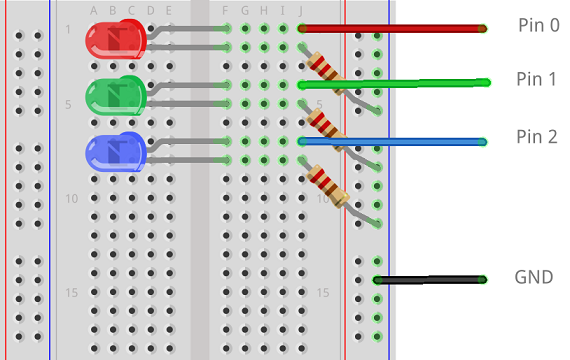# BBC micro:bit LEDs With MicroPython

## Introduction

You can use the external pins to connect more LEDs and have colours other than red. Using some LEDs gives us a chance to use the 3 main large pins as digital and analogue outputs.

This project uses 3 LEDs. Each LED will need a resistor. Here I've used 220 Ohm resistors, one for each of the LEDs used.

## Making The Circuit

The longer legs of the LEDs are the anodes or positive legs. These are the ones we connect to the micro:bit pins. The shorter legs are the cathodes, negative. They get connected to GND through a resistor.## Programming - Digital

We can turn the LEDs off by using the write_digital() method and supplying either a 1 (HIGH) or 0 (LOW) in the brackets to specify what we want to do.

This program blinks each of the LEDs in turn.

```from microbit import *
pin0.write_digital(0)
pin1.write_digital(0)
pin2.write_digital(0)
sleep(1000)
while True:
pin0.write_digital(1)
sleep(500)
pin0.write_digital(0)
pin1.write_digital(1)
sleep(500)
pin1.write_digital(0)
pin2.write_digital(1)
sleep(500)
pin2.write_digital(0)```

## Programming - Analog

We use the write_analog() with a value from 0 to 1023. This creates a PWM signal (pulse width modulation) on the pin that makes the LEDs fade in and out very slowly.

```from microbit import *
pin0.write_digital(0)
pin1.write_digital(0)
pin2.write_digital(0)
sleep(1000)
while True:
for i in range(0,1024):
pin0.write_analog(i)
pin1.write_analog(i)
pin2.write_analog(i)
sleep(10)
for i in range(1023,-1,-1):
pin0.write_analog(i)
pin1.write_analog(i)
pin2.write_analog(i)
sleep(10)```

## Challenge

Using 3 LEDs, you can count up to 7 in binary. The following table shows you how we can represent these using 3 bits (3 binary place values).

DenaryBinary
0000
1001
2010
3011
4100
5101
6110
7111

The following pseudocode will give you a one or zero in the variables fours, twos and units. Your leftmost LED is the fours LED, then twos, then units.

```denary ← any integer from 0 to 7 included fours ← denary // 4 remainder ← denary % 4 twos ← remainder // 2 units ← remainder % 2```